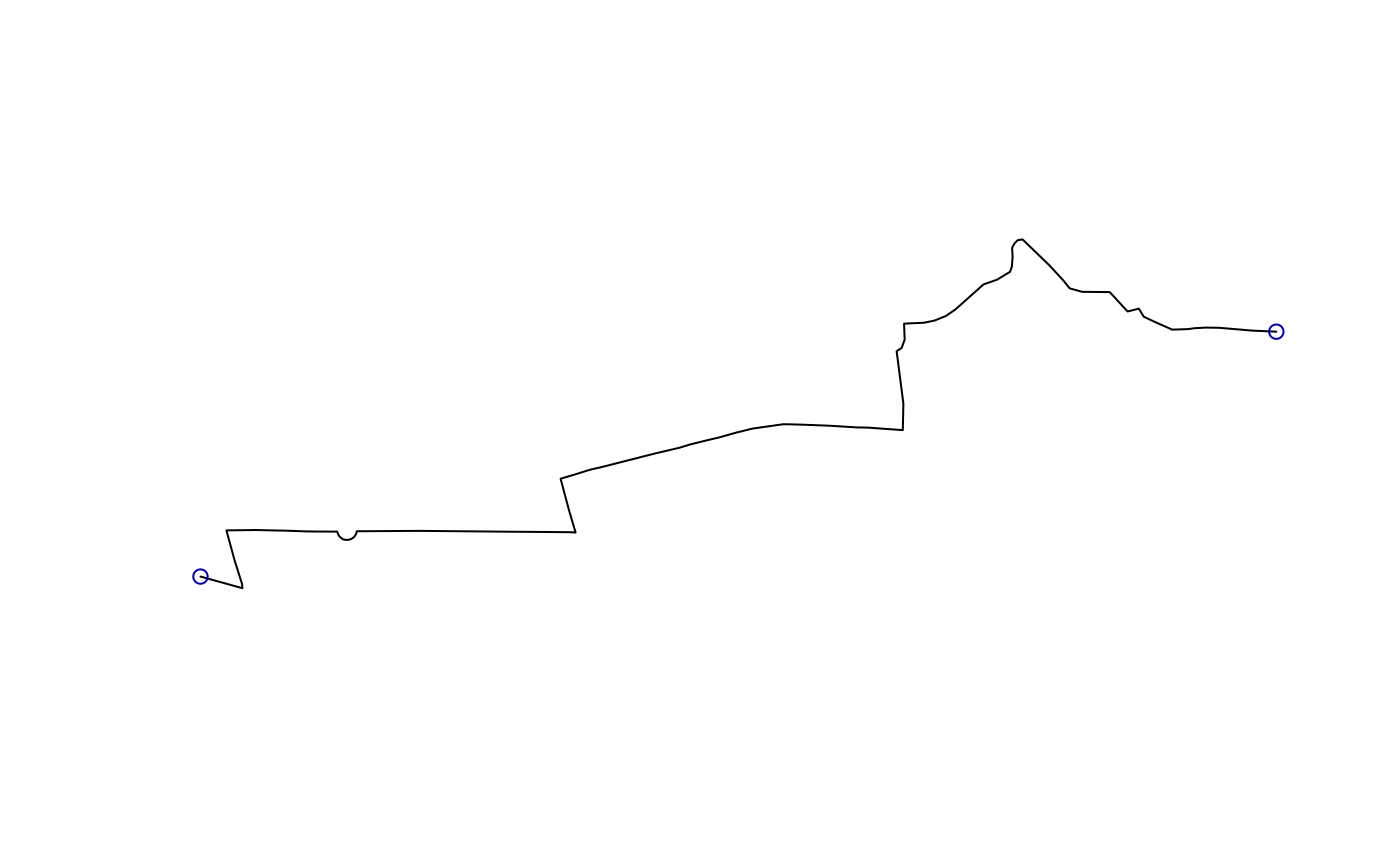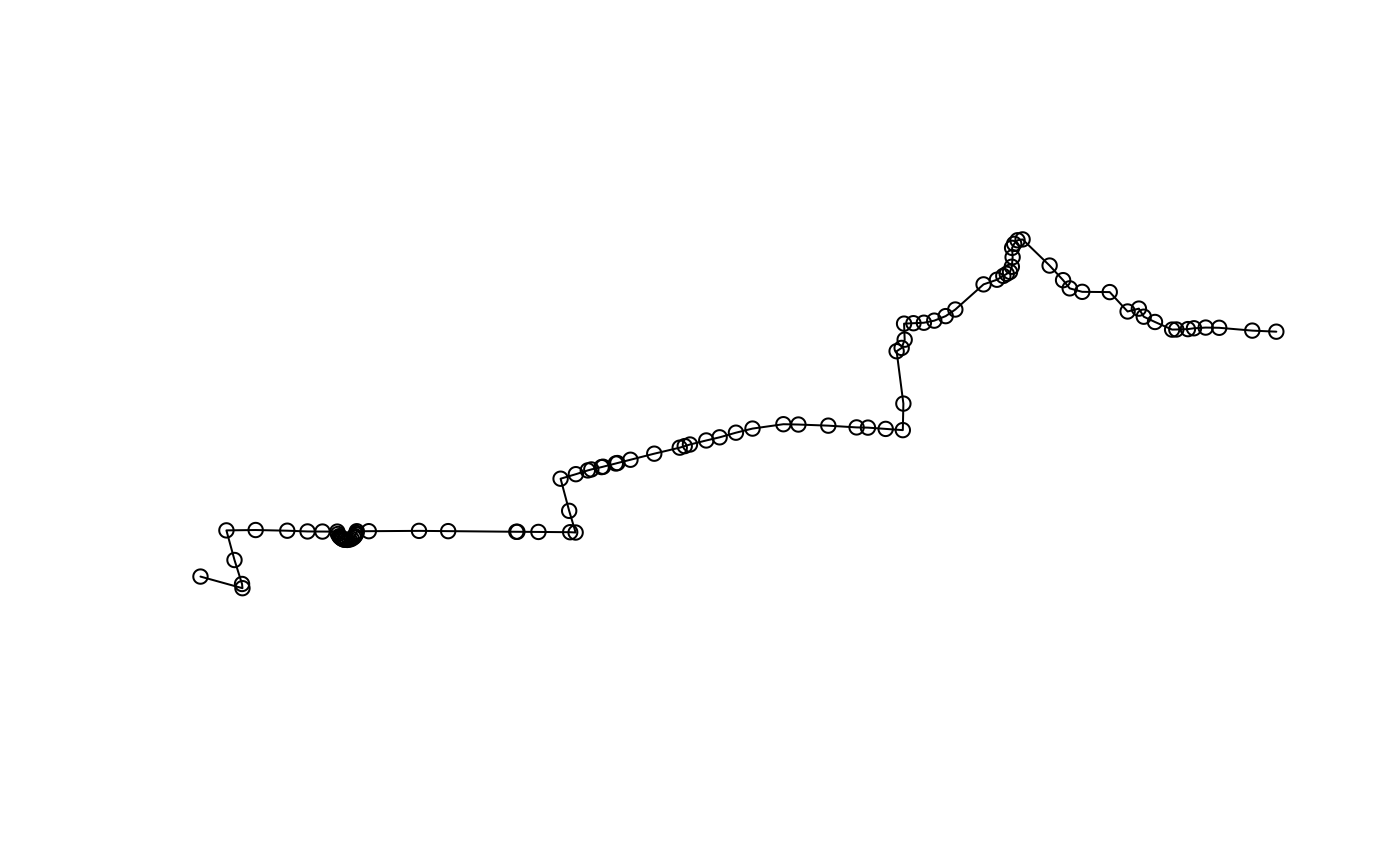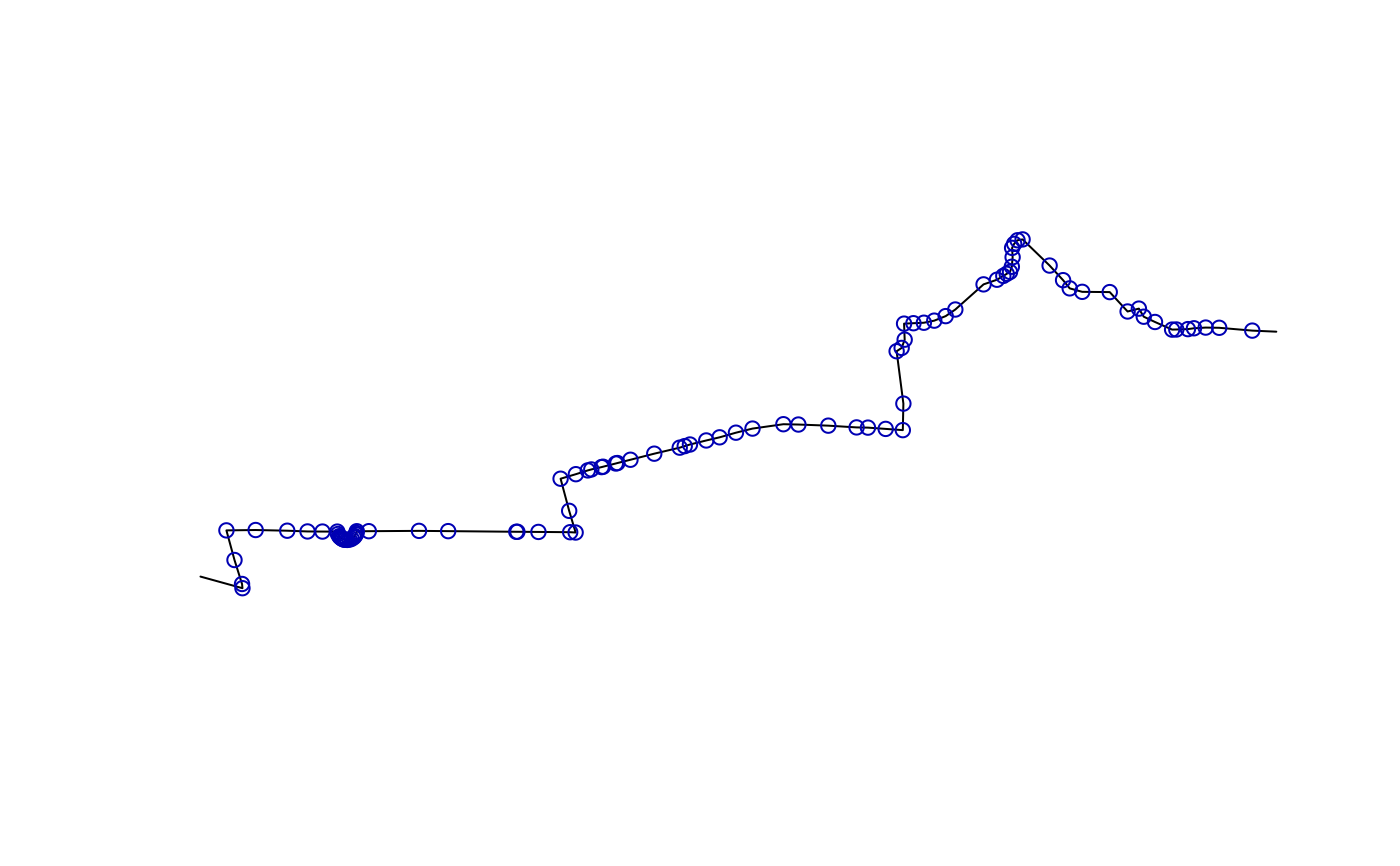The number of points will be double the number of lines with `line2points`. A closely related function, `line2pointsn` returns all the points that were line vertices. The points corresponding with a given line, `i`, will be `(2*i):((2*i)+1)`. The last function, `line2vertices`, returns all the points that are vertices but not nodes. If the input `l` object is composed by only 1 LINESTRING with 2 POINTS, then it returns an empty `sf` object.

## Usage

``````line2points(l, ids = rep(1:nrow(l)))

line2pointsn(l)

line2vertices(l)``````

## Arguments

l

An `sf` object or a `SpatialLinesDataFrame` from the older `sp` package

ids

Vector of ids (by default `1:nrow(l)`)

Other lines: `angle_diff()`, `geo_toptail()`, `is_linepoint()`, `line2df()`, `line_bearing()`, `line_breakup()`, `line_midpoint()`, `line_segment()`, `line_via()`, `mats2line()`, `n_vertices()`, `onewaygeo()`, `points2line()`, `toptail_buff()`

## Examples

``````l <- routes_fast_sf[2, ]
lpoints <- line2points(l)
plot(l\$geometry)# test all vertices:
plot(l\$geometry)
lpoints2 <- line2pointsn(l)